Instant Maths Help:

# Edexcel A Level

## Curve Sketching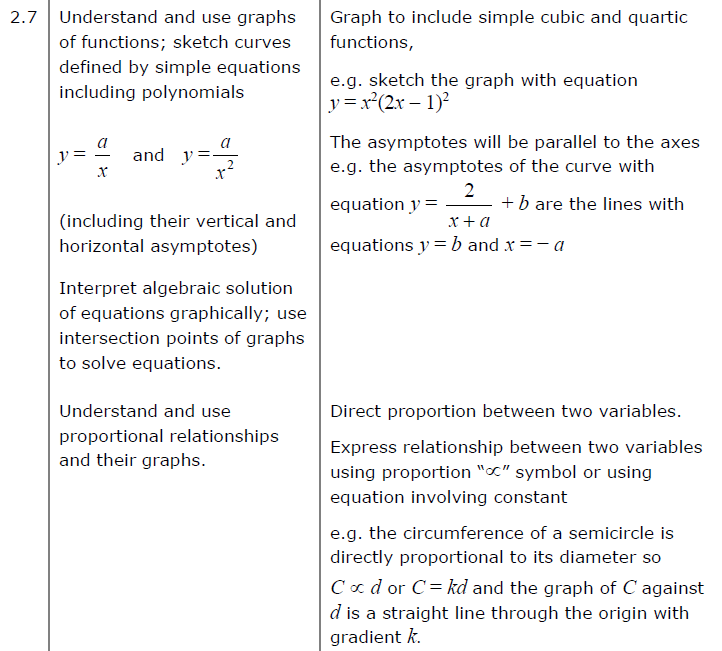##### Edexcel 8MA0/01 Jun 2023 AS Exam Q. 4 :   5 marks in 6:00 min.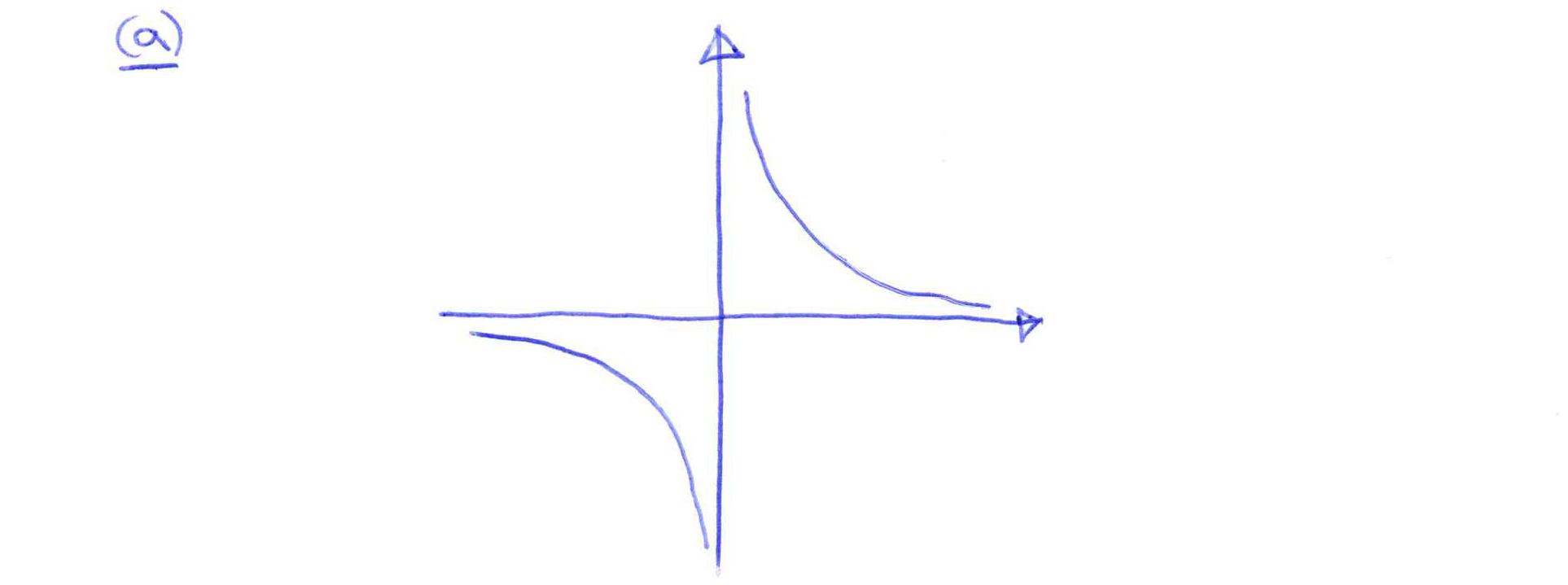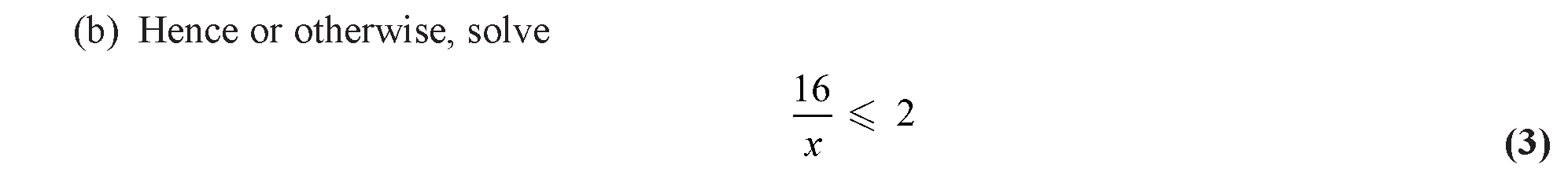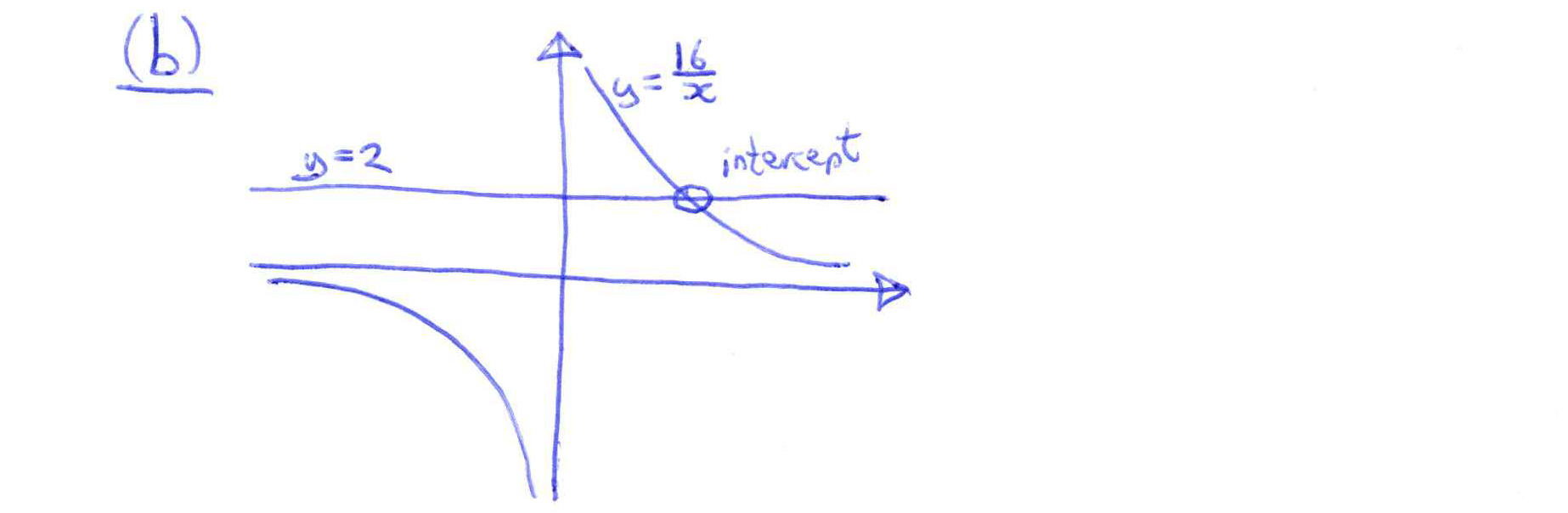##### Explore the roots and intercepts of cubic graphs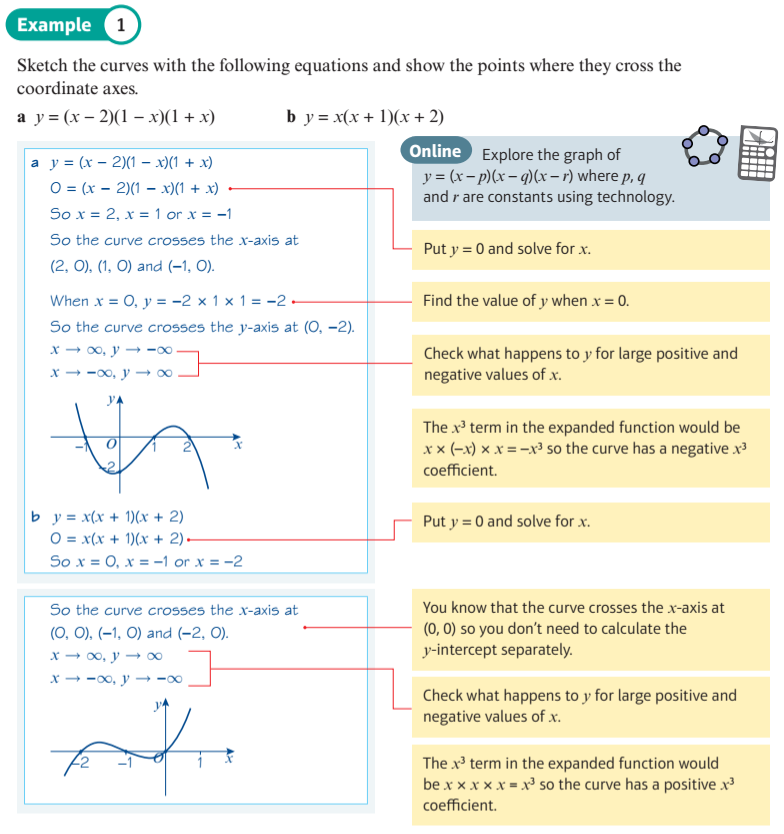##### Explore the roots and shapes of quartic functions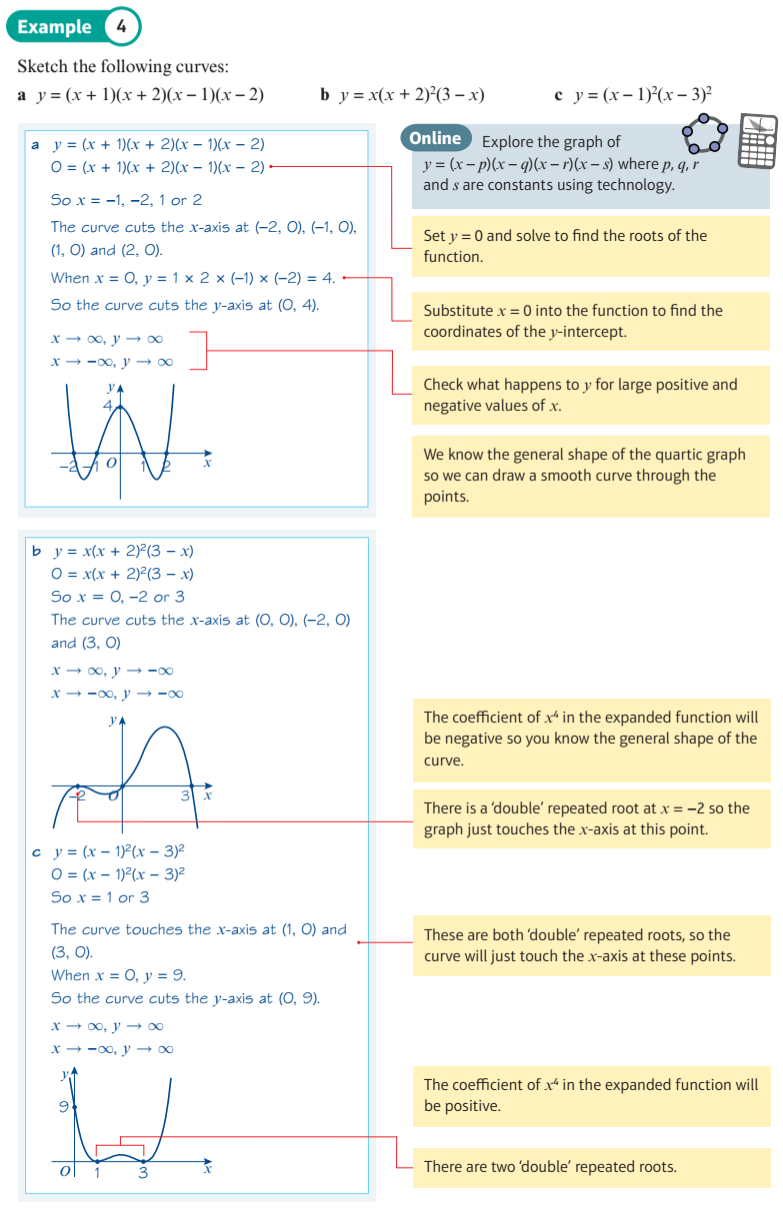##### Explore reciprocal graphs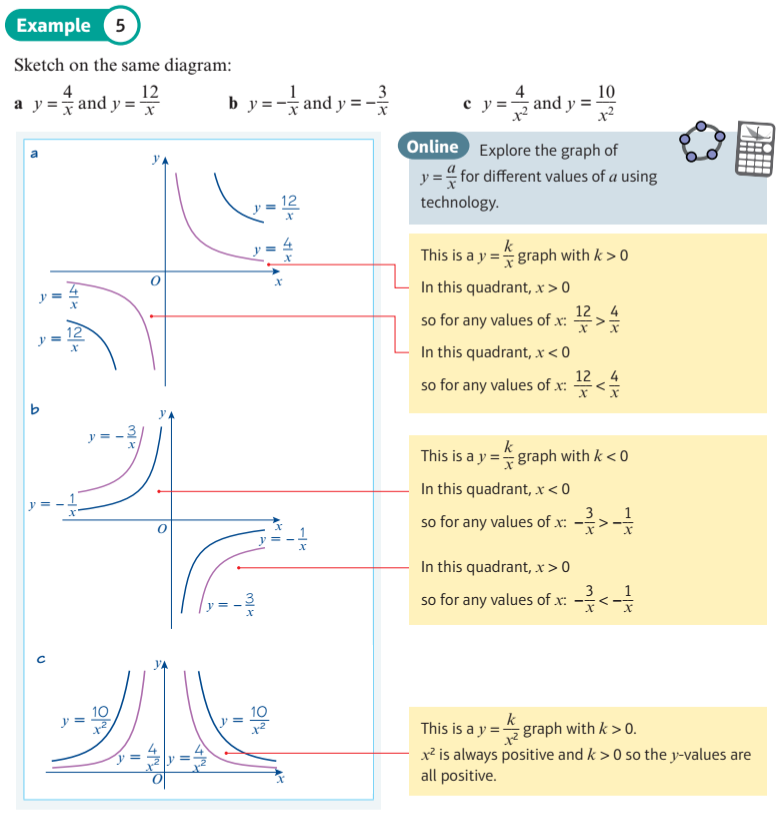##### Matching Polynomials
Selecting the order of the curve, and using the sliders to match the blue dashed curve to the green curve will reveal the equation in each case, but can you say what the equation is before making the match?
##### Coordinate Geometry - Rational Curves - y=(x-a)/(x-b)
The graph of $\color{blue}{y = { {x - a} \over {x - b} } }$

Change the values of $\color{blue}{a}$ and $\color{blue}{b}$ and observe the effect this has on the curve and its asymptotes.

##### Coordinate Geometry - Asymptotes - Asymptotes of y=a/(x-b)
An asymptote is a line towards which a given curve is approaching but never meets. Asymptotes are often vertical or horizontal lines, but can also be at an angle.

The graph displayed is described by the Cartesian equation: $\color{blue}{y = {{a} \over {x - b}}}$.

Change the values of $\color{blue}{a}$ and $\color{blue}{b}$ and observe the effect this has on the curve and its asymptotes. What are the equations of these asymptotes?
##### Odd and Even Functions
Functions with the property that f(-a) = f(a) for all values of a are called even.
Functions with the property that f(-a) = -f(a) for all values of a are called odd.
Drag the sample point to help decide whether each of the 10 functions given below are odd, even or neither.
What geometric properties do odd and even functions have?